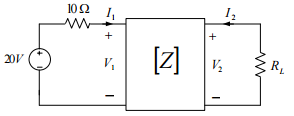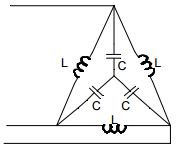# Gate Electrical Answer Key 7th Feb 2016

Q.34 The driving point input impedance seen from the source Vs of the circuit shown below, in Ω, is ______.Q.35 The z-parameters of the two port network shown in the figure are z11 =40 W , z12 = 60W z21 = 80 W and z22 = 100W . The average power delivered to RL = 20 W , in watts, is _______.Q.36 In the balanced 3-phase, 50 Hz, circuit shown below, the value of inductance (L) is 10 mH. The value of the capacitance (C) for which all the line currents are zero, in millifarads, is ___________.Q.37 In the circuit shown below, the initial capacitor voltage is 4 V. Switch S1 is closed at t=0. The charge (in µC) lost by the capacitor from t=25 μs to t=100 μs is ____________.Please report if you find any errors in the questions.
Keep watching the space←Previous
« Prev Post
Next→
Next Post »

#### 1 comment:

1.Q34 ans is 22 na?

Video Lectures